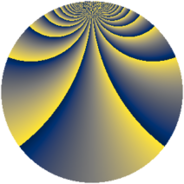# Properties

 Label 1045.2.ccLevel $1045$ Weight $2$ Character orbit 1045.cc Rep. character $\chi_{1045}(67,\cdot)$ Character field $\Q(\zeta_{36})$ Dimension $1200$ Sturm bound $240$

# Related objects

## Defining parameters

 Level: $$N$$ $$=$$ $$1045 = 5 \cdot 11 \cdot 19$$ Weight: $$k$$ $$=$$ $$2$$ Character orbit: $$[\chi]$$ $$=$$ 1045.cc (of order $$36$$ and degree $$12$$) Character conductor: $$\operatorname{cond}(\chi)$$ $$=$$ $$95$$ Character field: $$\Q(\zeta_{36})$$ Sturm bound: $$240$$

## Dimensions

The following table gives the dimensions of various subspaces of $$M_{2}(1045, [\chi])$$.

Total New Old
Modular forms 1488 1200 288
Cusp forms 1392 1200 192
Eisenstein series 96 0 96

## Trace form

 $$1200 q - 24 q^{6} + O(q^{10})$$ $$1200 q - 24 q^{6} - 36 q^{15} - 72 q^{16} + 36 q^{17} - 96 q^{21} - 24 q^{23} - 72 q^{28} - 72 q^{32} + 36 q^{35} - 96 q^{36} + 36 q^{38} - 72 q^{40} - 96 q^{43} - 36 q^{45} + 72 q^{47} - 168 q^{48} + 60 q^{57} - 144 q^{58} - 288 q^{60} + 48 q^{61} + 144 q^{63} - 180 q^{65} - 192 q^{72} - 96 q^{76} + 264 q^{78} + 252 q^{80} + 96 q^{81} - 300 q^{82} + 312 q^{86} + 420 q^{90} + 144 q^{91} + 276 q^{92} - 144 q^{93} + 72 q^{95} + 96 q^{96} + 72 q^{97} - 144 q^{98} + O(q^{100})$$

## Decomposition of $$S_{2}^{\mathrm{new}}(1045, [\chi])$$ into newform subspaces

The newforms in this space have not yet been added to the LMFDB.

## Decomposition of $$S_{2}^{\mathrm{old}}(1045, [\chi])$$ into lower level spaces

$$S_{2}^{\mathrm{old}}(1045, [\chi]) \cong$$ $$S_{2}^{\mathrm{new}}(95, [\chi])$$$$^{\oplus 2}$$